今日，上汽通用汽车雪佛兰品牌宣布探界者RS在2017成都国际车展强悍登场，共推三款车型，售价22.09万至25.09万元。探界者RS在探界者基础上升级打造，换装独有的黑色主题套件，以曜黑外观和RS专属标识彰显探界者RS更强悍、更科技、更时尚的产品实力。雪佛兰探界者RS的推出将有力扩充探界者产品阵容，也进一步满足敢于探索人生的“探界者”们更加个性化的消费需求。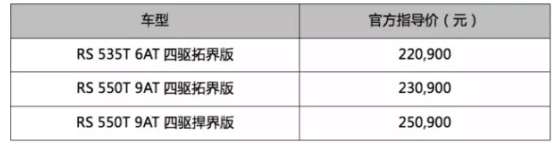（即日起至10月31日，消费者购买雪佛兰探界者全系车型可享价值30,000元的三重礼遇，包括万元购置税补贴、万元金融补贴和万元购车大礼包，零息首付仅需55,000元起！此外，购买探界者535T驰界版车型更可同步享受5,000元保险基金。）

*车身颜色：火山红、极夜黑

*内饰颜色：玄武黑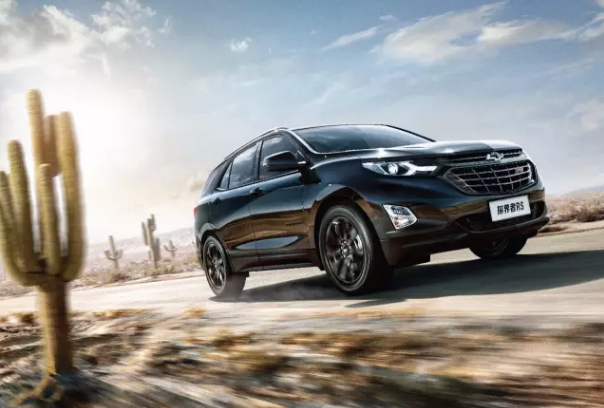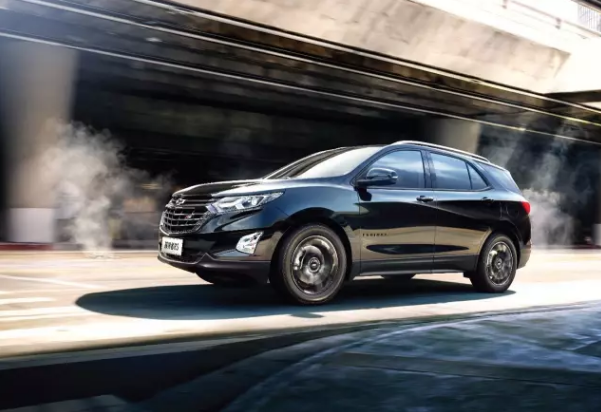作为家族最新成员，雪佛兰探界者RS凭借酷炫外观、高性能动力组合和丰富的科技配备，为消费者带来更强悍、更具力量感的驾驶体验。探界者RS车身线条硬朗而富有张力，车头、车尾配有RS专属耀红标识、LOGO“变身”雪佛兰黑色领结，对家族标志性的双格栅、外后视镜、全车铭牌、轮毂、门把手均做了特别黑色处理，用八大个性专属外观套件彰显探界者RS极具科技感和个性的运动气质。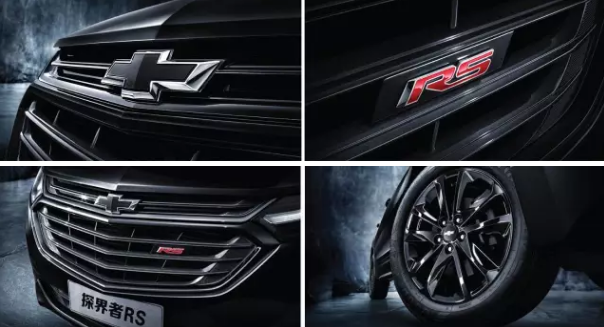在动力方面，基于通用汽车全球最新一代中级SUV平台打造的探界者RS，搭载2.0T双涡管直喷涡轮发动机匹配通用汽车首款9速HYDRA-MATIC®手自一体变速箱，以及通用汽车唯一一款高性能版1.5T顶置直喷涡轮发动机匹配6速DSS智能启停变速箱等两大动力组合，完美呈现高效强悍的驱动科技，并将SUV的驾控表现和燃油经济性提升至新的高度。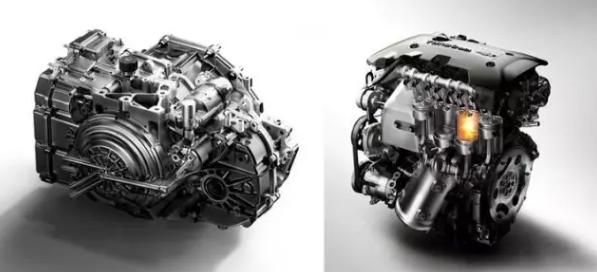2.0T+9AT动力组合完美呈现探界者高效强悍的驱动科技

探界者RS还搭载上汽通用最新的车载互联科技 – 新一代OnStar安吉星智能车联系统，能实现安全防护、移动设备互联、远程控制、语音交互等20余项功能，并全新开启了车载APP应用与服务。探界者RS同时也配备了兼容Apple CarPlay的8英寸触控中控大屏、BOSE剧院级高级音响、包含19项子系统的全功能电子安全系统以及10项同级领先智能安全系统等丰富人性化科技和智能安全配置，再加上传承雪佛兰SUV顶级安全标准的坚固车身结构和精益制造标准，成就探界者RS的非凡实力。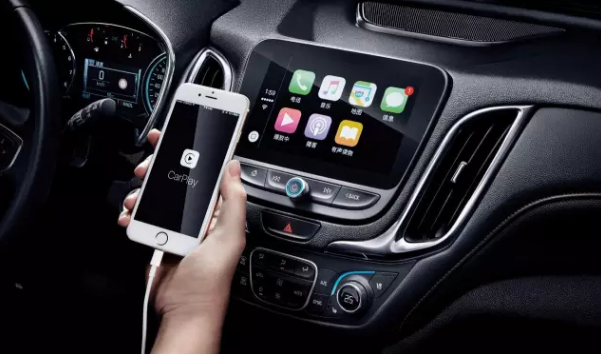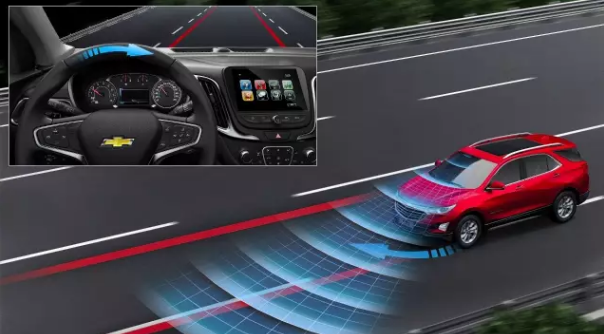自今年4月正式登陆中国以来，雪佛兰探界者便受到消费市场的广泛关注，新车上市45天即销量破万，截至7月底累计销售超过2.2万辆，其中550T车型销量占比达到25%-30%，充分体现了消费者对于探界者全新动力总成尤其是9AT变速箱的高度认可。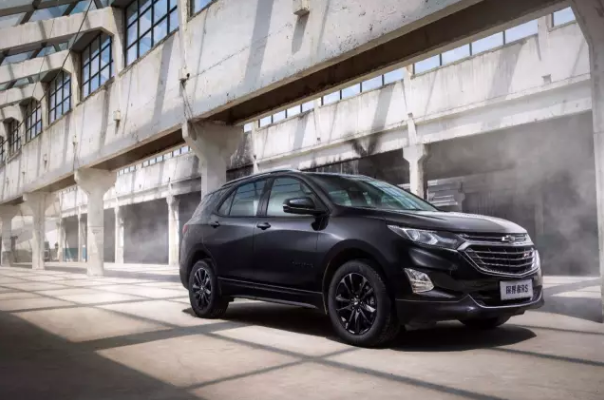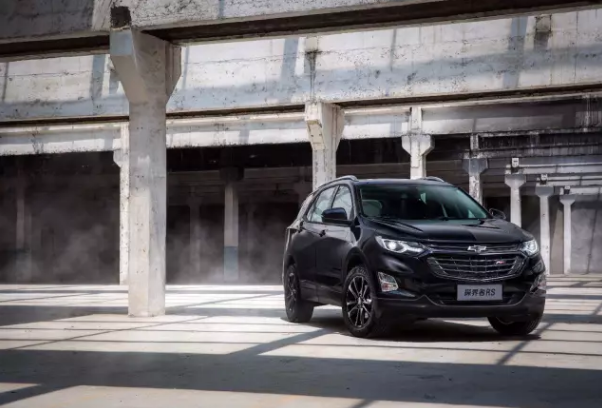为应对中国汽车市场新一轮的消费升级，雪佛兰积极制定前瞻布局和产品结构优化，并在18个月里完成主力产品与全球同步更新换代，形成了以第六代科迈罗、迈锐宝XL、全新科鲁兹等性能跑车与轿车构成的“高性能”产品线，以及由实力中级SUV探界者、全新创酷和皮卡“双子星”索罗德和库罗德所组成的“全功能”产品线，有效提升产品结构并推动整体销量的持续增长。探界者RS上市后将助力雪佛兰品牌在双元产品线的强势布局中，为寻求突破、渴望不同的年轻用户带去更多个性化的选择。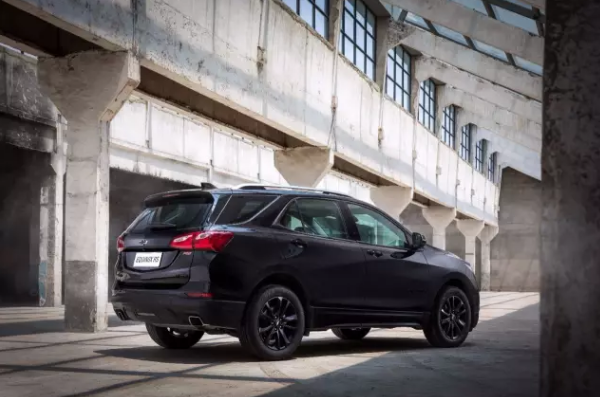雪佛兰探界者RS带来更强悍、更具力量感的驾驶体验

3号馆雪佛兰展台 全擎价到 浓烈美式风席卷展台

要玩儿嗨，独一无二印有你专属名字的百事限量“黑”罐等你报上名;真实的机甲互动体验“VR变装”,真人瞬间变装英勇大黄蜂;“遇见NASCAR”炫酷照片框,“小钢炮”科鲁兹合影帅酷炸;“X-box&LED”互动专区,体验肾上腺素飙升的快感;“3D赛车”比赛，拼得就是心跳！

有大礼，在展馆寻到雪佛兰礼仪热情红包就到手；展台扫码留资领卡并集齐5个游戏专区通关盖章；幸运抽奖可赢雪佛兰定制礼品，订车好礼享多重！电视、空调、iwatch、探界者定制手机壳……等精彩好礼接连来袭！

“雪佛兰中级实力SUV”探界者36期免息, 日供低至74元；“新一代旗舰轿车”迈锐宝XL36期免息，日供低至69元；“中高级车实力典范”迈锐宝36期免息，日供低至51 元；“新锐性能中级车”全新科鲁兹36期免息，日供低至44元；“新生代城市SUV”创酷24期免息，日供低至55 元；“精品全能家轿王”科沃兹24期免息，日供低至69元……

8月25日-9月3日，世纪城新国际会展中心3号馆雪佛兰展台，美式生活强风袭来，“探乐无界”展台见！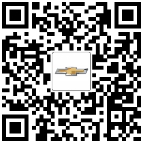##### 相关资讯

|分享

•已阅！握爪
•我手滑为你点赞
•128个赞！
•不明觉厉
•阅后既醉
•有钱！任性
•照片太美，我不敢看
•干货！杠杠的
•高大上
•膜拜中！
•请接受我的膝盖
•猫哥/猫妹么么哒
•神吐槽
•我只笑笑不说话
•我想静静
•窒息
##### 资讯相关车系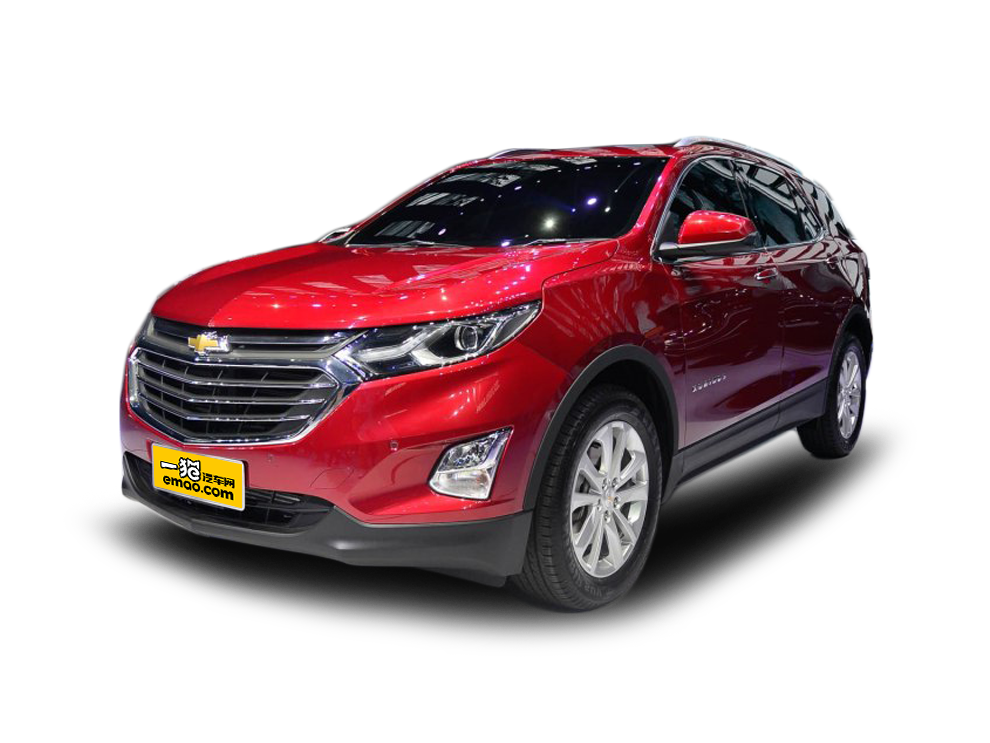##### 热度排行• 阿斯顿·马丁
• 奥迪
• 阿尔法罗密欧
• ALPINA

• 巴博斯
• 宝骏
• 宝马
• 保时捷
• 北汽制造
• 奔驰
• 奔腾
• 本田
• 比亚迪
• 标致
• 别克
• 宾利
• 布加迪
• 北汽威旺
• 北京
• 北汽绅宝
• 北汽幻速
• 北汽新能源
• 宝沃
• 比速汽车
• 北汽道达

• 昌河
• 长安
• 长城
• 长安商用
• 成功汽车
• 长江EV
• 长安轻车型

• 大众
• 道奇
• 东风
• 东风风神
• 东风小康
• 东南
• DS
• 东风风行
• 东风风度
• 东风风光

• 法拉利
• 菲亚特
• 丰田
• 福迪
• 福特
• 福田汽车
• 福汽启腾
• 风诺

• GMC
• 广汽传祺
• 广汽吉奥
• 观致

• 哈飞
• 海格
• 海马
• 华泰
• 黄海
• 恒天
• 红旗
• 哈弗
• 华颂
• 华凯
• 华泰新能源
• 汉腾汽车

• Jeep
• 江淮
• 江铃
• 捷豹
• 金杯
• 九龙
• 吉利汽车
• 金旅
• 金龙
• 江铃集团轻汽
• 江铃集团新能源
• 君马汽车
• 捷途

• 开瑞
• 凯迪拉克
• 科尼赛克
• 克莱斯勒
• KTM
• 卡威
• 凯翼
• 康迪
• 康迪电动汽车集团

• 猎豹汽车
• 兰博基尼
• 劳斯莱斯
• 雷克萨斯
• 雷诺
• 理念
• 力帆
• 莲花汽车
• 林肯
• 铃木
• 陆风
• 路虎
• 路特斯
• 领志
• 领克
• 零跑汽车

• MG
• MINI
• 马自达
• 玛莎拉蒂
• 迈凯伦
• 摩根

• 纳智捷

• 讴歌
• 欧宝
• 欧朗
• 欧拉
• 欧尚汽车

• 帕加尼

• 奇瑞
• 启辰
• 起亚
• 前途
• 庆铃汽车

• 日产
• 荣威
• 瑞麒汽车
• 如虎
• 瑞驰

• 上汽大通
• smart
• 三菱
• 双环
• 双龙
• 斯巴鲁
• 斯柯达
• 萨博
• 思铭
• 赛麟
• SWM斯威汽车

• TESLA
• 泰卡特
• 腾势

• 威麟
• 威兹曼
• 沃尔沃
• 五菱汽车
• 五十铃
• 潍柴英致
• WEY
• 蔚来
• 威马汽车

• 现代
• 雪佛兰
• 雪铁龙
• 西雅特
• 新特汽车
• 小鹏汽车
• 新宝骏

• 野马汽车
• 一汽
• 依维柯
• 英菲尼迪
• 永源
• 驭胜

• 中华
• 中兴
• 众泰
• 知豆
• 之诺
• 正道汽车
• A
• B
• C
• D
• E
• F
• G
• H
• I
• J
• K
• L
• M
• N
• O
• P
• Q
• R
• S
• T
• U
• V
• W
• X
• Y
• Z

• 阿斯顿·马丁
• 奥迪
• 阿尔法罗密欧
• ALPINA

• 巴博斯
• 宝骏
• 宝马
• 保时捷
• 北汽制造
• 奔驰
• 奔腾
• 本田
• 比亚迪
• 标致
• 别克
• 宾利
• 布加迪
• 北汽威旺
• 北京
• 北汽绅宝
• 北汽幻速
• 北汽新能源
• 宝沃
• 比速汽车
• 北汽道达

• 昌河
• 长安
• 长城
• 长安商用
• 成功汽车
• 长江EV
• 长安轻车型

• 大众
• 道奇
• 东风
• 东风风神
• 东风小康
• 东南
• DS
• 东风风行
• 东风风度
• 东风风光

• 法拉利
• 菲亚特
• 丰田
• 福迪
• 福特
• 福田汽车
• 福汽启腾
• 风诺

• GMC
• 广汽传祺
• 广汽吉奥
• 观致

• 哈飞
• 海格
• 海马
• 华泰
• 黄海
• 恒天
• 红旗
• 哈弗
• 华颂
• 华凯
• 华泰新能源
• 汉腾汽车

• Jeep
• 江淮
• 江铃
• 捷豹
• 金杯
• 九龙
• 吉利汽车
• 金旅
• 金龙
• 江铃集团轻汽
• 江铃集团新能源
• 君马汽车
• 捷途

• 开瑞
• 凯迪拉克
• 科尼赛克
• 克莱斯勒
• KTM
• 卡威
• 凯翼
• 康迪
• 康迪电动汽车集团

• 猎豹汽车
• 兰博基尼
• 劳斯莱斯
• 雷克萨斯
• 雷诺
• 理念
• 力帆
• 莲花汽车
• 林肯
• 铃木
• 陆风
• 路虎
• 路特斯
• 领志
• 领克
• 零跑汽车

• MG
• MINI
• 马自达
• 玛莎拉蒂
• 迈凯伦
• 摩根

• 纳智捷

• 讴歌
• 欧宝
• 欧朗
• 欧拉
• 欧尚汽车

• 帕加尼

• 奇瑞
• 启辰
• 起亚
• 前途
• 庆铃汽车

• 日产
• 荣威
• 瑞麒汽车
• 如虎
• 瑞驰

• 上汽大通
• smart
• 三菱
• 双环
• 双龙
• 斯巴鲁
• 斯柯达
• 萨博
• 思铭
• 赛麟
• SWM斯威汽车

• TESLA
• 泰卡特
• 腾势

• 威麟
• 威兹曼
• 沃尔沃
• 五菱汽车
• 五十铃
• 潍柴英致
• WEY
• 蔚来
• 威马汽车

• 现代
• 雪佛兰
• 雪铁龙
• 西雅特
• 新特汽车
• 小鹏汽车
• 新宝骏

• 野马汽车
• 一汽
• 依维柯
• 英菲尼迪
• 永源
• 驭胜

• 中华
• 中兴
• 众泰
• 知豆
• 之诺
• 正道汽车
• A
• B
• C
• D
• E
• F
• G
• H
• I
• J
• K
• L
• M
• N
• O
• P
• Q
• R
• S
• T
• U
• V
• W
• X
• Y
• Z

• 阿斯顿·马丁
• 奥迪
• 阿尔法罗密欧
• ALPINA

• 巴博斯
• 宝骏
• 宝马
• 保时捷
• 北汽制造
• 奔驰
• 奔腾
• 本田
• 比亚迪
• 标致
• 别克
• 宾利
• 布加迪
• 北汽威旺
• 北京
• 北汽绅宝
• 北汽幻速
• 北汽新能源
• 宝沃
• 比速汽车
• 北汽道达

• 昌河
• 长安
• 长城
• 长安商用
• 成功汽车
• 长江EV
• 长安轻车型

• 大众
• 道奇
• 东风
• 东风风神
• 东风小康
• 东南
• DS
• 东风风行
• 东风风度
• 东风风光

• 法拉利
• 菲亚特
• 丰田
• 福迪
• 福特
• 福田汽车
• 福汽启腾
• 风诺

• GMC
• 广汽传祺
• 广汽吉奥
• 观致

• 哈飞
• 海格
• 海马
• 华泰
• 黄海
• 恒天
• 红旗
• 哈弗
• 华颂
• 华凯
• 华泰新能源
• 汉腾汽车

• Jeep
• 江淮
• 江铃
• 捷豹
• 金杯
• 九龙
• 吉利汽车
• 金旅
• 金龙
• 江铃集团轻汽
• 江铃集团新能源
• 君马汽车
• 捷途

• 开瑞
• 凯迪拉克
• 科尼赛克
• 克莱斯勒
• KTM
• 卡威
• 凯翼
• 康迪
• 康迪电动汽车集团

• 猎豹汽车
• 兰博基尼
• 劳斯莱斯
• 雷克萨斯
• 雷诺
• 理念
• 力帆
• 莲花汽车
• 林肯
• 铃木
• 陆风
• 路虎
• 路特斯
• 领志
• 领克
• 零跑汽车

• MG
• MINI
• 马自达
• 玛莎拉蒂
• 迈凯伦
• 摩根

• 纳智捷

• 讴歌
• 欧宝
• 欧朗
• 欧拉
• 欧尚汽车

• 帕加尼

• 奇瑞
• 启辰
• 起亚
• 前途
• 庆铃汽车

• 日产
• 荣威
• 瑞麒汽车
• 如虎
• 瑞驰

• 上汽大通
• smart
• 三菱
• 双环
• 双龙
• 斯巴鲁
• 斯柯达
• 萨博
• 思铭
• 赛麟
• SWM斯威汽车

• TESLA
• 泰卡特
• 腾势

• 威麟
• 威兹曼
• 沃尔沃
• 五菱汽车
• 五十铃
• 潍柴英致
• WEY
• 蔚来
• 威马汽车

• 现代
• 雪佛兰
• 雪铁龙
• 西雅特
• 新特汽车
• 小鹏汽车
• 新宝骏

• 野马汽车
• 一汽
• 依维柯
• 英菲尼迪
• 永源
• 驭胜

• 中华
• 中兴
• 众泰
• 知豆
• 之诺
• 正道汽车
• A
• B
• C
• D
• E
• F
• G
• H
• I
• J
• K
• L
• M
• N
• O
• P
• Q
• R
• S
• T
• U
• V
• W
• X
• Y
• Z